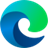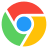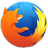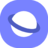# scale() CSS Function

### Description

The CSS `scale()` function is used to resize an element on the 2D plane. It takes one or two values as arguments, representing the amount of scaling to be applied in each direction.

If one value is given, the element is scaled the same amount in both the X and Y directions. If two values are given, the element is scaled in the X and Y directions independently.

The scaling values can be specified as numbers or percentages. A number value represents a factor by which the element is scaled. A percentage value represents a percentage of the element's original size.

For example, the following CSS code will scale an element to twice its original size:

``````.element {
transform: scale(2);
}
``````

The following CSS code will scale an element to half its original size in the X direction and twice its original size in the Y direction:

``````.element {
transform: scale(0.5, 2);
}
``````

The `scale()` function can be used to create a variety of effects, such as resizing images, making text larger or smaller, and creating zoom animations.

Here are some examples of how the `scale()` function can be used:

``````/* Resize an image to twice its original size */
img {
transform: scale(2);
}

/* Make all text on a page 20% larger */
body {
font-size: 120%;
}

/* Create a zoom animation */
.element {
transition: transform 0.5s;
}

.element:hover {
transform: scale(1.2);
}
``````

### Syntax

```transform: scale(<numberX> [, <numberY>]?);
/* the question mark indicates the second value is optional */```

### Values

• <numberX>The scale of the element horizontally.
• <numberY>The scale of the item vertically. If no value is specified, it defaults to <numberX>.

### Example

``<img class="scale" src="images/nature.jpg" alt="Nature Image">``
``````.scale {
transform: scale(2, 1);
}``````

### Browser Support

The following table will show you the current browser support for the CSS `scale()` function.

 Desktop12 1 3.5 10.1 3.5
 Tablets / Mobile18 4 11 3.2 1 2

Last updated by CSSPortal on: 7th October 2023